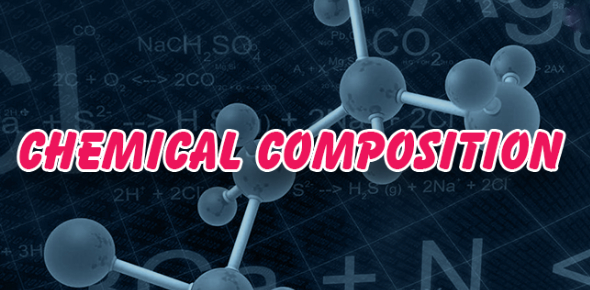# Cement Quiz: Chemical Compositions!

Approved & Edited by ProProfs Editorial Team
At ProProfs Quizzes, our dedicated in-house team of experts takes pride in their work. With a sharp eye for detail, they meticulously review each quiz. This ensures that every quiz, taken by over 100 million users, meets our standards of accuracy, clarity, and engagement.
| Written by Thinkeasy
T
Thinkeasy
Community Contributor
Quizzes Created: 1 | Total Attempts: 251
Questions: 10 | Attempts: 251SettingsHey nerdy superhero! Here we have got a short quiz for you, it's all about cement and the chemical compositions behind it. So, what are you waiting for? Go ahead, and spend some time in this quiz below.

• 1.

### Lime content in cement is

• A.

22%

• B.

62%

• C.

15%

• D.

3%

B. 62%
Explanation
As cement is a calcareous material.

Rate this question:

• 2.

### Calcium sulphate is a

• A.

Accelarator

• B.

Retarder

• C.

Reduce initial setting time

• D.

None

B. Retarder
Explanation
Calcium sulphate is commonly known as gypsum, which is used as a retarder in various industries. As a retarder, it slows down the setting time of materials such as cement, allowing for better workability and longer working time. Gypsum is often added to cement or concrete mixes to control the setting time and prevent rapid hardening. Therefore, the correct answer for this question is "Retarder".

Rate this question:

• 3.

• A.

Aelite

• B.

Celite

• C.

Belite

• D.

Felite

B. Celite
• 4.

### Heat of hydration of Opc at 7 days is 90 to 100 cal/gm

• A.

True

• B.

False

B. False
Explanation
The given statement is false. The heat of hydration of OPC (Ordinary Portland Cement) at 7 days is not 90 to 100 cal/gm. The heat of hydration refers to the heat released during the chemical reaction between cement and water, which leads to the hardening of concrete. The specific value for the heat of hydration can vary depending on factors such as the type of cement, water-cement ratio, and curing conditions.

Rate this question:

• 5.

### Mass specific gravity of cement is 3.15

• A.

True

• B.

False

B. False
Explanation
Mass specific gravity is 1.5 and true specific gravity is 3.15

Rate this question:

• 6.

### Specific gravity of cement is done by le chatlier flask

• A.

True

• B.

False

A. True
Explanation
The specific gravity of cement is indeed determined using a Le Chatelier flask. This device is specifically designed to measure the specific gravity of materials, including cement. It works by measuring the displacement of a liquid when a sample of the material is placed in the flask. By comparing the weight of the displaced liquid to the weight of an equal volume of water, the specific gravity of the cement can be calculated. Therefore, the statement that the specific gravity of cement is determined using a Le Chatelier flask is true.

Rate this question:

• 7.

### For finess test in sieve the content should not more than 5% for rapid hardening cement.

• A.

True

• B.

False

A. True
Explanation
The statement is true because during the fineness test in sieve, the content should not exceed 5% for rapid hardening cement. This means that the cement particles should be finely ground and have a maximum particle size of 45 microns. If the content exceeds 5%, it indicates that the cement is not finely ground and may have a coarse texture, which can affect its performance and strength. Therefore, to ensure the quality and effectiveness of rapid hardening cement, it is important to meet the fineness requirement during the sieve test.

Rate this question:

• 8.

### Ratio of compressive strength of cylinder to cube is

• A.

1.2

• B.

0.92

• C.

1.5

• D.

2

B. 0.92
Explanation
The ratio of compressive strength of a cylinder to a cube is given as 0.92. This means that the compressive strength of a cylinder is 0.92 times the compressive strength of a cube. In other words, the cube has a higher compressive strength compared to the cylinder. This ratio is important in engineering and construction as it helps determine the strength and stability of different structures.

Rate this question:

• 9.

### Initial setting time of high alumina cement

• A.

2 hour

• B.

3.5 hour

• C.

5 hour

• D.

7 hour

B. 3.5 hour
Explanation
The correct answer is 3.5 hours. The initial setting time of high alumina cement refers to the time it takes for the cement to start hardening after it is mixed with water. In this case, it takes approximately 3.5 hours for the high alumina cement to reach its initial setting point, at which it becomes solid and can no longer be easily manipulated.

Rate this question:

• 10.

### Total loss on ignition should not be more than 4%

• A.

True

• B.

FalseBack to top